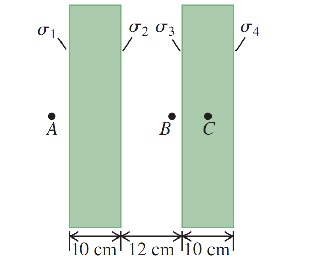Question
88 views

Two very large, nonconducting plastic sheets, each 10.0 cm thick, carry uniform charge densities σ 1 , σ 2 , σ 3 and σ 4 on their surfaces, as shown in the following figure(Figure 1). These surface charge densities have the values σ 1 = -7.70 μC/ m^2 , σ 2 =5.00μC/ m^2 , σ 3 = 2.70 μC/ m^2 , and σ 4 =4.00μC/ m^2 . Use Gauss's law to find the magnitude and direction of the electric field at the following points, far from the edges of these sheets.

Part A What is the magnitude of the electric field at point A , 5.00 cm from the left face of the left-hand sheet? Express your answer to three significant figures and include the appropriate units.

Part B What is the direction of the electric field at point A , 5.00 cm from the left face of the left-hand sheet?

Part C What is the magnitude of the electric field at point B , 1.25 cm from the inner surface of the right-hand sheet? Express your answer to three significant figures and include the appropriate units.

Part D What is the direction of the electric field at point B, 1.25 cm from the inner surface of the right-hand sheet?

Part E
What is the magnitude of the electric field at point C, in the middle of the right-hand sheet?
Express your answer to three significant figures and include the appropriate units.

Part F
What is the direction of the electric field at point C, in the middle of the right-hand sheet?help_outlineImage Transcriptionclose/ A Ko 10 cm 12 cm 10 cm fullscreen
check_circle

Step 1

Part A:

Step 2

Part B: Since, the magnitude in part A is negative, direction is towards the left.

Step 3

Part C:...

### Want to see the full answer?

See Solution

#### Want to see this answer and more?

Solutions are written by subject experts who are available 24/7. Questions are typically answered within 1 hour.*

See Solution
*Response times may vary by subject and question.
Tagged in

### Electric Charges and Fields